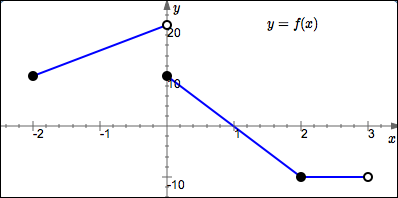﻿ Reading Information from the Graph of a Function

#Reading Information from the Graph of a Function

Suggested Review:

You must be able to read a wide variety of information from the graph of a function:

## Example

The graph of a function $\,f\,$ is given below.$f(0) = 10$

$f(1) = 0$

$f(2.03) = -10$

$\text{dom}(f) = [-2,3)$

$\text{ran}(f) = [-10,20)$

$f$ increases on $[-2,0)$

$f$ decreases on $[0,2]$

$f$ is constant on $[2,3)$

$\{x\ |\ f(x) = 10\} = \{-2,0\}$

$\{t\ |\ f(t) \gt 0\} = [-2,1)$

How can we tell this is the graph of a function?   It passes the vertical line test.

## Concept Practice

In the graphs, all black points are plotted on grid lines. That is, both the $x$-values and $y$-values are integers.

For green points, you're only guaranteed that the $x$-value is an integer. Round any computed $y$-values to three decimal points (as needed).